# Speeding up quiz

#### Quiz Description

This quiz will be focusing on acceleration and velocity. In our day-to-day life, we experience cars in motion, when these cars tend to move at a faster rate as their motion proceeds, it is known as speeding up. In the course of taking this quiz, you will learn things like velocity, acceleration, instantaneous speed, and all the laws of motion. GCE, GCSE, and SAT exams are often set from this particular part of Physics as it is Mechanics with Newton's laws. Therefore taking the quiz will help you study and have a better view of speeding up in order to pass your upcoming exams.

Speeding up is the rate of change of velocity with time. In other words, it is defined as acceleration. Speeding up is governed by laws, known as Newton's laws of motion. There are 3 of these laws and they help us in the calculation of velocity, and acceleration when given a problem to solve. These laws can be summarized mathematically as

V^2=U^2+at.................(1)

V2=U^2+2aS ...................(2)

S=Ut+1/2at^2 ..................(3)

For an object in motion; Where V is the final speed of the object, U is the initial speed of the object, a is acceleration, t is the time of motion, and S is the distance covered throughout the motion

Quizzes here are meant to open your mind wider, and these Physics quizzes are very much technical and so require precision, and taking the quiz will help you get that.
Do not forget these are exam standard quizzes with options one to four with only one being correct.
Good Luck!

Which of the following matches a speed of 12.5m/s?

Correct
•  a car moves 400m in 36s
•  a conveyer belt moves along 20m in 2.5s
•  a cyclist covers 500m in 40s
•  a sprinter runs 100m in 10.2s

Which of the following matches a speed of 11.1m/s?

Correct
•  a cyclist covers 500m in 40s
•  a car moves 400m in 36s
• a sprinter runs 100m in 10.2s
•   a conveyer belt moves along 20m in 2.5s

A child is playing on a slide. Which statement is NOT true?

Correct
• the two main forces involved are weight due to gravity and friction acting in opposite directions
•  when the force of friction equals the child's weight, the downward speed decreases
•  if the child sat on a rough cushion the 'slide' will be slower
•  if the child tucks in their arms and head the 'slide' will be faster

When a lift is operating, the two main forces are the tension in the cable, from the motor action, and the weight of the lift car and people, due to gravity. Which of the following applies to the situation when the lift is moving down and slowing down? (e.g. as lift reaches bottom)

Correct
•   the tension is less than the weight
•   the tension is equal to the weight
•  the tension is more than the weight
• None

A car travels 40 km in 30 minutes. What is the average speed of the car?

Correct
•  120 km/hour
•   1.33 km/hour
•   40 km/hour
•  80 km/hour

A train travels 40 km in 20 minutes. What is the average speed of the train?

Correct
•  120 km/hour
• 80 km/hour
• 2 km/hour
•   20 km/hour

An athlete completes a 400 m race in 50 seconds. What is the average speed of the athlete?

Correct
• 10 m/s
•  0.125 m/s
•  9 m/s
•  8 m/s

Which of the following is a unit of speed?

Correct
• m
• m/s
• N
• s

When a lift is operating, the two main forces are the tension in the cable, from the motor action, and the weight of the lift car and people, due to gravity. Which of the following applies to the situation when the lift is moving upwards and slowing down? (e.g. as lift reaches top)

Correct
• the tension is equal to the weight
•  the tension is more than the weight
• the tension is less than the weight
• None

A cyclist is travelling at an average speed of 5 m/s. How far will the cyclist travel in 10 s?

Correct
•   0.5 m
• 500 m
•  2 m
•  50 m

What is speed of the cyclist after 32 seconds?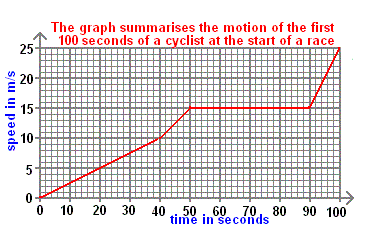Correct
•  8 m/s
•  7 m/s
•  6 m/s
• 10 m/s

The graph shows part of an urban car journey in terms of the distance the car has travelled in a certain time. What is the average speed of the car from 40 to 80 seconds?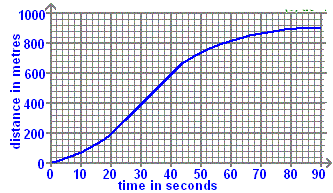Correct
•  15.0 m/s
•  12.5 m/s
• 7.5 m/s
•  0.0 m/s

Which diagram (?) corresponds to when the acceleration is the greatest?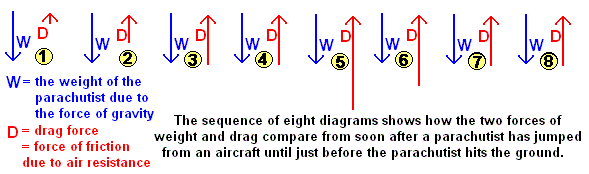Correct
• diagram (3)
•  diagram (5)
•  diagram (7)
•  diagram (1)

The graph shows the comparative distance-time behaviour of a 500kg object dropped on the Earth, Jupiter and the Earth's Moon from a height of 20 000m. Some 'drops' may involve the use of a parachute. Which d-t graph line corresponds to dropping the object on Jupiter?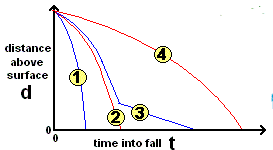Correct
• graph line (3)
•  graph line (2)
• graph line (4)
•  graph line (1)

The graph shows the comparative distance-time behaviour of a 500kg object dropped on the Earth, Jupiter and the Earth's Moon from a height of 20 000m. Some 'drops' may involve the use of a parachute. Which d-t graph line corresponds to dropping the object on the Moon with a parachute?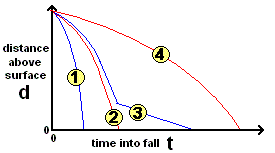Correct
• graph line (2)
•  graph line (4)
•  graph line (1)
•  graph line (3)